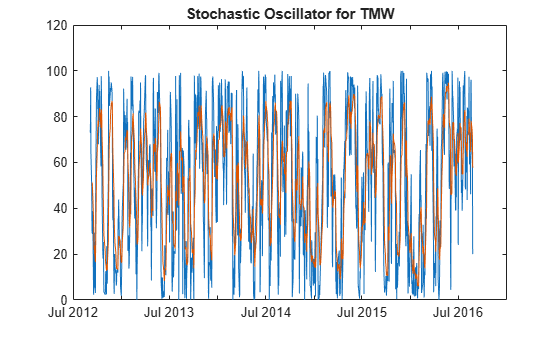Documentation

stochosc

Stochastic oscillator

stochosc has been partially removed and will no longer accept a fints object (tsobj) argument. Use a matrix, timetable, or table instead for financial time series.

Use fts2timetable to convert a fints object to a timetable object.

Description

example

percentKnD = stochosc(Data) calculates the stochastic oscillator.

example

percentKnD = stochosc(___,Name,Value) adds optional name-value pair arguments.

Input Arguments

collapse all

Data with high, low, open, close information, specified as a matrix, table, or timetable. For matrix input, Data is an M-by-3 matrix of high, low, and closing prices stored in the corresponding columns, respectively. Timetables and tables with M rows must contain variables named 'High', 'Low', and 'Close' (case insensitive).

Data Types: double | table | timetable

Name-Value Pair Arguments

Specify optional comma-separated pairs of Name,Value arguments. Name is the argument name and Value is the corresponding value. Name must appear inside quotes. You can specify several name and value pair arguments in any order as Name1,Value1,...,NameN,ValueN.

Example: percentKnD = stochosc(TMW,'NumPeriodsD',10,'NumPeriodsK',3,'Type','exponential')

Period difference for PercentK, specified as the comma-separated pair consisting of 'NumPeriodsK' and a scalar positive integer.

Data Types: double

Length of moving average in periods for PercentD, specified as the comma-separated pair consisting of 'NumPeriodsD' and a scalar positive integer.

Data Types: double

Moving average method for PercentD calculation, specified as the comma-separated pair consisting of 'Type' and a character vector with a value of:

• 'exponential' – Exponential moving average is a weighted moving average. Exponential moving averages reduce the lag by applying more weight to recent prices. For example, a 10 period exponential moving average weights the most recent price by 18.18%.

• 'triangular' – Triangular moving average is a double-smoothing of the data. The first simple moving average is calculated and then a second simple moving average is calculated on the first moving average with the same window size.

Data Types: char

Output Arguments

collapse all

PercentK and PercentD, returned with the same number of rows (M) and type (matrix, table, or timetable) as the input Data.

Examples

collapse all

Load the file SimulatedStock.mat, which provides a timetable (TMW) for financial data for TMW stock.

oscillator = stochosc(TMW,'NumPeriodsD',7,'NumPeriodsK',10,'Type','exponential');
plot(oscillator.Time,oscillator.FastPercentK,oscillator.Time,oscillator.FastPercentD)
title('Stochastic Oscillator for TMW')collapse all

Stochastic Oscillator

The stochastic oscillator calculates the Fast PercentK (F%K), Fast PercentD (F%D), Slow PercentK (S%K), and Slow PercentD (S%D) from the series of high, low, and closing stock prices.

By default, the stochastic oscillator is based on 10-period difference for PercentK and a 3-period exponential moving average for PercentD.

 Achelis, S. B. Technical Analysis from A to Z. Second Edition. McGraw-Hill, 1995, pp. 268–271.